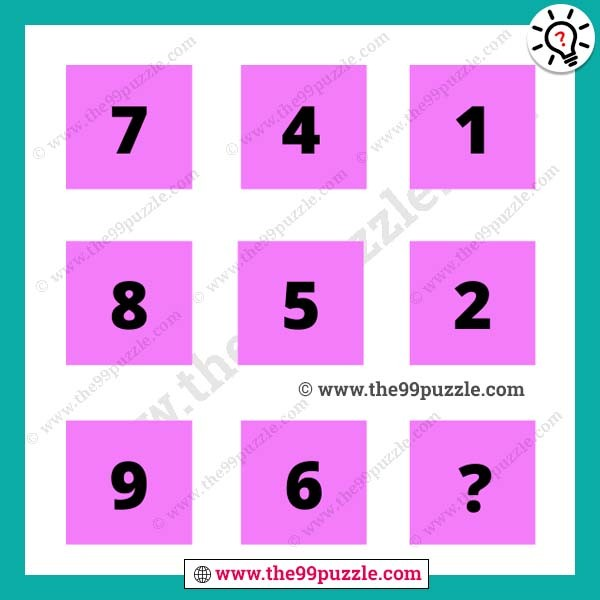# Find missing number logic puzzle with answer – Puzz242

Find missing number logic puzzle for tricky mind people. These box puzzles are asked in many competitive exams and interviews. These are the logical math number puzzles that can crack your logical mind. Now many teachers ask their students to solve this mathematics reasoning. Always you can try to solve these brain teaser puzzles that help you to calculate these equations quickly. In this puzzle picture, you see numbers in the box logically. If you are a genius at puzzles and riddles you solve this quickly.###### Explanation:

1st column = (7+9)÷2 = 8

2nd column = (4+6)÷2 = 5

3rd column = (1+3)÷2 = 2